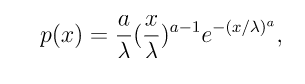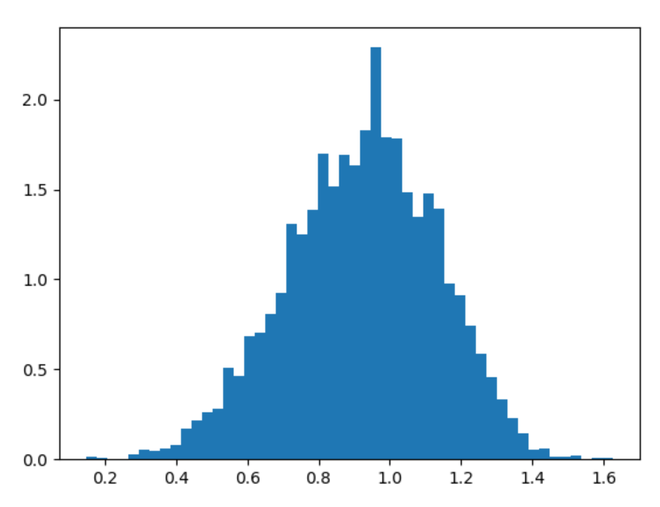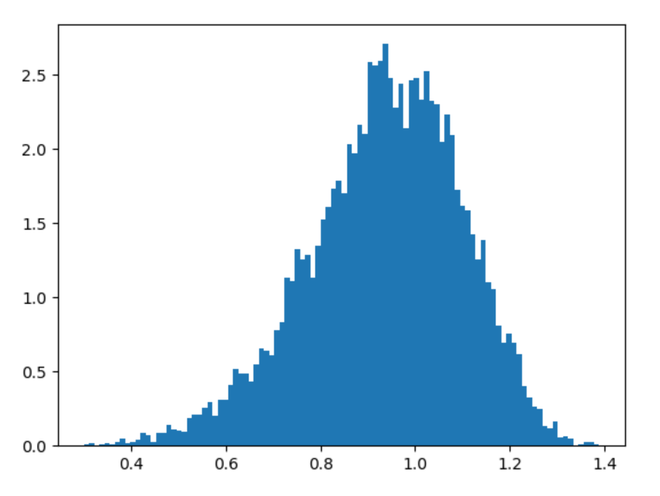Related Articles

# numpy.random.weibull() in Python

• Last Updated : 18 Aug, 2020

With the help of numpy.random.weibull() method, we can get the random samples from weibull distribution and return the random samples as numpy array by using this method.Weibull Distribution

Syntax : numpy.random.weibull(a, size=None)

Return : Return the random samples as numpy array.

Example #1 :

In this example we can see that by using numpy.random.weibull() method, we are able to get the random samples from weibull distribution and return the numpy array.

## Python3

 `# import numpy``import` `numpy as np``import` `matplotlib.pyplot as plt`` ` `# Using weibull() method``gfg ``=` `np.random.weibull(``5``, ``5000``)`` ` `plt.hist(gfg, bins ``=` `50``, density ``=` `True``)``plt.show()`

Output :Example #2 :

## Python3

 `# import numpy``import` `numpy as np``import` `matplotlib.pyplot as plt`` ` `# Using weibull() method``gfg ``=` `np.random.weibull(``6.78``, ``10000``)`` ` `plt.hist(gfg, bins ``=` `100``, density ``=` `True``)``plt.show()`

Output :Attention geek! Strengthen your foundations with the Python Programming Foundation Course and learn the basics.

To begin with, your interview preparations Enhance your Data Structures concepts with the Python DS Course. And to begin with your Machine Learning Journey, join the Machine Learning – Basic Level Course

My Personal Notes arrow_drop_up